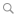BlogMatlab syntax tricks

By Raphaël - December 18th, 2016

Matlab

0 Comment

Structures

# Arrays

## Pushing at the end of an array with end+1

Here is a basic exemple:

% Define an empty array
A = [];                     % A is empty

% Push a value at the end
A(end+1) = 18;              % A now contains 1 element
A(end+1) = 19;              % A now contains 2 element
A(end+1) = 20;              % A now contains 3 elements

>> A

A =

18    19    20

This syntax is particularly useful to fill up an array at every iteration of a loop. Note that it is generally considered a sub-optimal syntax and pre-allocation should be used as a golden rule whenever possible:

Pre-allocation exemple
% Pre-allocate the array
A = NaN();

% Fill the array within a loop
for i = 1:100
A(i) = randi(i);
end

Note that though the offical documentation generally pre-allocate arrays with zeros, pre-allocating with NaNs is generally a better pratice as it is easier to debug incorrectly assigned values.

But there are cases where the size of the resulting array cannot be predicted before the loop begins. Pre-allocation is then suboptimal, and one has to increment the size of the array whenever a new element has to be inserted; in these cases the end+1 syntax is useful. For instance:

% Define an empty array
A = [];

for i = 1:100

% Some random condition that makes the final array size unpredictable
%   Here: wait a random fraction of a second, get the number of seconds
%   in the current time and check if it is above the number of minutes.

pause(rand(1));
tmp = clock;
if tmp(end)>tmp(end-1)

% Extend the array size and assign the value
A(end+1) = i;

end

end

Finally, it is also possible to use the end+1 syntax with multi-dimensional arrays if the initial array dimensions are correctly defined. Remember you can define a dimension with zero cardinality:

% Define an empty array
A = NaN(0,2);             % A is a 0-by-2 empty array

N = 100;
for i = 1:N

% Some random condition that makes the final array size unpredictable
%   Here: draw a random number below the current index and compare it
%   against a random number below the loop size.

r = i*rand(1);
if r>N*rand(1)

% Extend the array size and memorize iteration index and value
A(end+1,:) = [i r];

end

## Deleting array elements

To remove an element (or a set of elements) from an array, the elegant way simply writes:

A(I) = [];

where I denotes the indexes of the elements to remove.

In the case of multiple-elements deletion, this syntax is definitely the most efficient and elegant way. But note that for removing a single element the =[] syntax is twice as slower than redefining the array with concatenation:

% Define an array
A = 1:10;

% Array concatenation
A = [A(1:4) A(6:end)];      % Takes ~4.3e-6 seconds on my laptop

% Direct deletion
A(5) = [];                  % Takes ~8.9e-6 seconds, twice as slow!

## Using strings as arrays

In Matlab, strings are arrays of chars and actually behave like any other array. For instance one can use the same concatenation syntax in all dimensions:

>> s = ['abc' 'def' 'ghi']

s =

abcdefghi

>> s = ['Hello' ; 'world']

s =

Hello
world

Indexing also works the same:

>> s = 'Hello world !';   % Define a string
>> s(7:11)                % Select a substring

ans =

world

>> s(s=='o') = '*'        % Replace all occurence of 'o' by '*'

s =

Hell* w*rld !

>> s(1:2:end) = '#'       % Replace one every two letters

s =

#e#l# #*#l# #